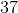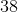# Common Core: 1st Grade Math : Understanding Place Value

## Example Questions

### Example Question #1031 : Common Core Math: Grade 1

What digit is in the tens place?Explanation:

The tens place is always the second number from the right.

### Example Question #1032 : Common Core Math: Grade 1

What digit is in the tens place?Explanation:

The tens place is always the second number from the right.

### Example Question #1033 : Common Core Math: Grade 1

What digit is in the tens place?Explanation:

The tens place is always the second number from the right.

### Example Question #1034 : Common Core Math: Grade 1

What digit is in the tens place?Explanation:

The tens place is always the second number from the right.

### Example Question #1035 : Common Core Math: Grade 1

What digit is in the tens place?Explanation:

The tens place is always the second number from the right.

### Example Question #1036 : Common Core Math: Grade 1

What digit is in the tens place?Explanation:

The tens place is always the second number from the right.

### Example Question #1037 : Common Core Math: Grade 1

What digit is in the tens place?Explanation:

The tens place is always the second number from the right.

### Example Question #1038 : Common Core Math: Grade 1

What digit is in the tens place?Explanation:

The tens place is always the second number from the right.

### Example Question #1041 : Common Core Math: Grade 1

What digit is in the tens place?Explanation:

The tens place is always the second number from the right.

### Example Question #1042 : Common Core Math: Grade 1

What digit is in the tens place?